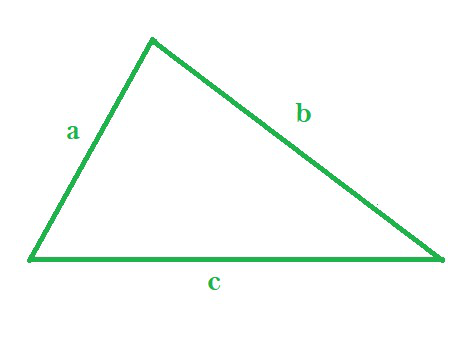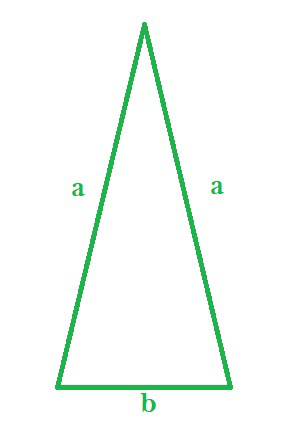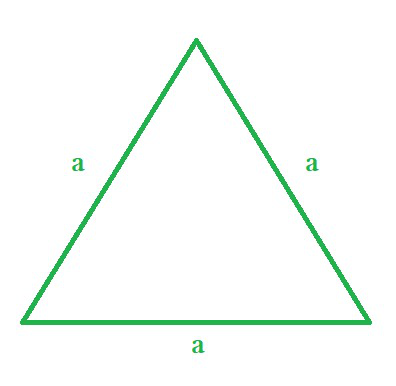# Perimeter of a Triangle Formula

• Last Updated : 21 Dec, 2021

Triangles are three-sided closed polygons formed by the intersection of three lines. It is encountered a lot in everyday life. It is one of the basic shapes of geometry. It has three sides, three angles, and three vertices. The sum of the three angles is always equal to 180°.

Types of Triangles

Triangles are divided into three types based on the sides of the triangle:

• Equilateral triangle: It is defined as a triangle whose all three sides are equal. All the angles of an equilateral triangle are equal to 60°.
• Isosceles triangle: It is defined as a triangle whose two sides are equal in length. Since an equilateral triangle also has any two sides equal, it can be classified as an isosceles triangle. An isosceles triangle has two equal angles at vertices opposite to its equal sides.
• Scalene triangle: It is defined as a triangle whose all three sides are different in length. Thus, the angles also have different measures.

Properties of Triangles

• It has three sides, which may be of the same or different lengths.
• The Sum of the two sides is always greater than the third side.
• The difference between the two sides is always smaller than the third side.
• The sum of all three angles of a triangle is equal to 180o.
• The Sum of two internal angles is equal to the external angle of the third side.

### Perimeter of a Scalene Triangle

The perimeter of a triangle is equal to the sum of all sides of a triangle. If the sides of a triangle have lengths equal to a, b, & c, then,A triangle with sides a, b and c

Perimeter = a + b + c

where,

a, b, c are the side lengths

### Perimeter of an Isosceles Triangle

For an isosceles triangle, if two equal sides are of length ‘a’ units and the length of unequal side equals to ‘b’, then,An isosceles triangle with sides ‘a’, ‘a’, and ‘b’

Perimeter of Isosceles triangle = a + a + b = 2a + b

where,

a is the length of two equal sides

b is the third side

### Perimeter of an Equilateral Triangle

For an equilateral triangle, since all sides are equal in length, thus a = b = c, henceAn equilateral triangle with all sides equal to ‘a’ units

Perimeter of equilateral triangle = a + a + a = 3a

where,

a is the side length

### Sample Questions

Question 1. If the length of sides of a triangle are 4cm, 5cm, and 6cm, then what is the perimeter of the triangle?

Solution:

Given, the sides of the triangle are 4cm, 5cm, and 6cm. Thus, it is an scalene triangle.

So the perimeter of the triangle  = Sum of sides = 4 + 5 + 6 = 15cm

Question 2. In an isosceles triangle, the two sides are given as 5cm and 6cm. What can be the perimeter of the triangle?

Solution:

Given, the triangle is the isosceles triangle. Thus, any two sides of the triangle are equal.

So, the third side can be either 5cm or 6cm.

If the third side is 5cm, then,

Perimeter = 5 + 5 + 6 = 16cm

If the third side is 6cm, then,

Perimeter = 5 + 6 + 6 = 17cm

So, the perimeter can be either 16cm or 17cm.

Question 3. What is the perimeter of an equilateral triangle whose one side length is 5cm?

Solution:

Given that the triangle is an equilateral triangle, thus all three sides are equal in length.

Since one side is equal to 5cm, the other two sides will also be equal to 5cm.

So, Perimeter = 5 + 5 + 5 = 15cm.

Question 4: Given the perimeter of an equilateral triangle is 21cm, find the length of its three sides.

Solution:

Since, in an equilateral triangle, all the three sides are equal in length, the perimeter is equal to three times the length of a side.

Let’s the length of any side be equal to ‘a’ units. So perimeter is equal to ‘3a’ units.

So, we can write,

3a = 21

a = 7cm

Thus, the length of each side is equal to 7cm.

Question 5. Find the length of two equal sides of an isosceles triangle if the length of the unequal side is 5cm and the perimeter is 17cm.

Solution:

Given, the length of unequal side is 5cm and perimeter is 17cm.

Since, it is isosceles triangle, length of other two sides are equal. Let each equal side length be ‘a’ units.

Thus, perimeter = a + a + 5

Since, perimeter = 17cm, we can write,

17 = a + a + 5

2a + 5 = 17

2a = 12

a = 6cm

Thus, the length of the equal sides of the isosceles triangle is 6cm.

My Personal Notes arrow_drop_up